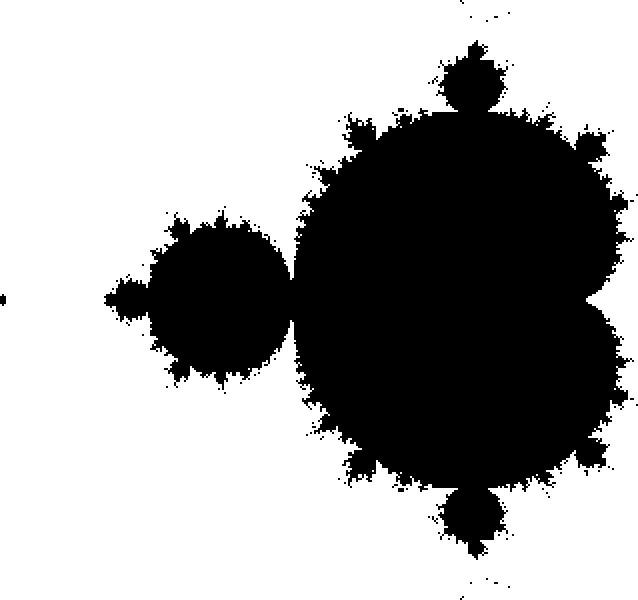We recently saw that through the power of hooks and forks, we could read J expressions just like we’d read an English sentence. But the expressive power of hooks and forks doesn’t end there. We can also write J expressions just like we’d speak them!

Let’s take a minute to refresh ourselves on how J’s hooks and forks work, and then see how we can use them to write some truly grammatical code.

## Forks and Hooks

If you think back to our last conversation about J, you’ll remember that we defined the `mean` verb to be a fork composed of three separate verbs: “sum” (`+/`), “divided by” (`%`), and “tally” (`#`).

All together, these three verbs form a fork that calculates the average of any list passed into it:

``````   mean =: +/ % #
mean 1 2 3 4
2.5
``````

When passed a single argument, the fork will apply that argument to each of the outer verbs monadically (passing them a single argument), and dyadically apply (passing two arguments) those results to the middle verb.

If you squint your eyes enough, this might start looking like a fork.

Similarly, we can create a hook by composing any two verbs together. For example, we can write an `append_mean` verb that forms a hook out of the “append” (`,`) verb and our new `mean` verb:

``````   append_mean =: , mean
``````

When passed a single argument, the fork monadically applies that argument to the right-hand verb, and dyadically applies the original argument and the result of the first verb to the left-hand argument.

Unfortunately no amount of squinting will make this look like a real-life hook.

The inner workings of J’s hooks and forks may seem a little complicated, but that low-level obfuscation leads to higher levels of clarity. Namely, we can read our hooks and forks like English sentences!

## Writing with Hooks and Forks

Not only do hooks and forks allow us to read our J expressions like English sentences, they also let us write our expressions like we’d write English sentences!

Let’s consider an example.

Imagine we want to construct the Mandelbrot set using J. To test whether a complex number belongs to the set, we repeatedly apply an iterative function to it. If the result of that function ever diverges to infinity (or exceeds a magnitude of two), the point does not belong to the set. If the number hasn’t diverged after some number of iterations, we can assume that it belongs to the set.The Mandelbrot set equation.

In terms of implementing this in J, it sounds like our best bet will be to store the result of each iteration in an array of complex numbers. The first element in the array will be the point under test (skipping `z₀` for convenience), and the last element will be the result of the last application of our iteration function. With that in mind, we can write a verb that computes our next iteration, given our list of `z` values.

``````   next =: {. + *:@:{:
``````

This expression is saying that `next` “is” (`=:`) the “first element of the array” (`{.`) “plus” (`+`) the “square of the last element of the array” (`*:@:{:`). That last verb combines the “square” (`*:`) and “last” (`{:`) verbs together with the “at” (`@:`) adverb.

You’ll notice that `next` is a fork. The argument application rules follow the structure we discussed above, but we’re able to read and write the expression linearly from left to write.

While we’re able to compute the next iteration of our Mandelbrot set equation, we still need to append the result back onto our list of `z` values. In plain English, we want to “append” (`,`) the “next” (`next`) value:

``````   append_next =: , next
``````

Just like our earlier example, `append_next` is a hook.

We can repeatedly apply our `append_next` verb to some initial value:

``````   append_next append_next append_next 0.2j0.2
0.2j0.2 0.2j0.28 0.1616j0.312 0.128771j0.300838
``````

Or we can do that more concisely with the “power” (`^:`) verb:

``````   (append_next^:3) 0.2j0.2
0.2j0.2 0.2j0.28 0.1616j0.312 0.128771j0.300838
``````

Perfect!

## Final Touches

As a final piece of magic to top off this exploration into J, let’s apply our `apply_next` verb repeatedly over a grid of complex numbers:

``````   axis =: 0.005 * 250 - (i.501)
grid =: (*&0j1 +/ |.) axis
mbrot =: (append_next^:40)"0 (grid - 0.6)
``````

We’re left with a three dimensional array of complex numbers in our `mbrot` noun, representing the values of `z` resulting from repeatedly applying our `append_next` function to each point in our grid of complex numbers.

As we mentioned earlier, if any of these values of `z` exceed a magnitude of `2`, we can assume they diverge and aren’t a part of the Mandelbrot set.

We can compute that divergence test and render the resulting using `viewmat`:

``````   viewmat <&2 | ({:"1 mbrot)
``````

And we’re left with a pretty picture of the Mandelbrot set!## Final Thoughts

It’s no joke that J can be an intimidating language to work with. I’m still trying to lift myself over the initial learning curve, and some of the expressions written above still take some concentration on my part to grok their meaning (even though I wrote them).

That said, there are some incredibly unique and interesting ideas baked into this language, and I’m getting a lot out of the learning experience.